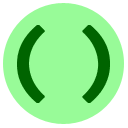# 15B: Python Pushups

Exercises 15A, 15B, and 15C can be completed in any order.

In this lesson, we give several medium-size exercises combining tools learned in earlier lessons.Coding Exercise: Forty Below In The Winter
In this exercise, you will create a temperature converter which will convert Fahrenheit values to Celsius and vice-versa. You will need the following two formulas which relate the temperature f in Fahrenheit to the temperature c in Celsius:$\displaystyle{f = c \times \frac{9}{5} + 32}$$\displaystyle{c = (f -32)\times\frac{5}{9}.}$

The input will be a string consisting of a floating-point number followed immediately by the letter F or C, such as "13.2C". You should convert to the other temperature scale and print the converted value in the same format. For example, if the input is "8F" then the output should be (approximately) "-13.333C", and if the input is "12.5C" then the output should be "54.5F".

You may enter input for the program in the box below.Coding Exercise: Credit Check
You have been hired by MeisterCard to write a function which checks if a given credit card number is valid. Your function check(S) should take a string S as input. First, if the string does not follow the format "#### #### #### ####" where each # is a digit, then it should return False. Then, if the sum of the digits is divisible by 10 (a "checksum" method), then the procedure should return True, else it should return False. For example, if S is the string "9384 3495 3297 0123" then although the format is correct, the digit sum is 72 so you should return False.
Enter testing statements like print(myfunction("test argument")) below.

In the next exercise, use the methods string.split(), which removes the spaces from a word and returns a list of the words it contains, and string.lower() which converts a string to lower case. For example,

• "Split these words!".split() returns the list ["Split", "these", "words!"]
• "LOWERCase".lower() returns "lowercase"

Note: split() can accept further options to split in other ways; see the documentation.Coding Exercise: Poetic Analysis
A writer is working on their newest poem, Turing and the Machines. They have hired you to determine the word which appears the most times. You can access the lines of the poem by calling input() repeatedly, and the last line contains the three characters ###. All lines consist of words separated by single spaces; there are no digits or punctuation. Convert all the words to lower-case, and print the word that occurs the most times (we guarantee there will not be a tie). For example, if the input is

Here is a line like sparkling wineLine up fast or be the last###
Then the output should be

line
since it appears twice and no other word appears twice.
You may enter input for the program in the box below.

Suppose you have n flavours of ice cream, and want to make a sundae using exactly k of those flavours. How many different flavour combinations are possible? For example, if n=4 and k=2, there are 6 possibilities:

(1) A and B, (2) A and C, (3) A and D, (4) B and C, (5) B and D, (6) C and D.

(for example here the flavours are Apricot, Blueberry, Chocolate, and Date).

Similarly, you could be choosing 2 people out of 4 (Al, Bob, Carol, Di) to make a committee. How many ways can the committee be built? The answer would still be 6. The next problem is about computing this number.Coding Exercise: Be Choosy
The number of combinations of k things out of a total of n things is equal to$\displaystyle{\frac{n}{k}\times\frac{n-1}{k-1}\times\frac{n-2}{k-2}\times\cdots\times\frac{n-k+2}{2}\times\frac{n-k+1}{1}}$

Write a function choose(n, k) which takes two integers n and k; we guarantee n>k>0. The function should return the value given in the formula above.
Note that for this exercise, we expect you to just mechanically compute this formula without trying to understand it. But if you're interested, here's a sample YouTube video explaining it.

Enter testing statements like print(myfunction("test argument")) below.

In mathematics, the number you computed in the previous exercise is usually written$\displaystyle{\binom{n}{k}}$

and called "n choose k." There are many interesting facts about these values such as$\displaystyle{\binom{n}{k} = \binom{n}{n-k}}$ and$\displaystyle{\binom{n}{k} = \binom{n-1}{k} + \binom{n-1}{k-1}}$

This is the end of our exercise session; you can move on to another lesson.